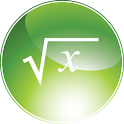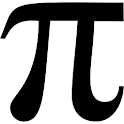Text Options for the Visually Impaired Font Size: Color:

# Math Technology Tools

### Quick Link to Technology Tool pages by subject:

An online scientific calculator. It doesn’t graph like a TI-84 but it can solve problems and functions.Choose any level math, from kindergarten to college, and race against your friends.Wolfram Alpha is a computational knowledge engine that uses of expert-level knowledge to compute answers to questions you ask.Use math to defeat the evil-doers of Magical Math World.Math Playground is a fun website for children to go to for assistance in learning basic math!XA site dedicated to helping students learn multiplication skills.Math is Fun

Math explained in easy language, plus puzzles, games, quizzes, worksheets and a forum. For K-12 kids, teachers and parents.Math.com

Math.com is dedicated to providing revolutionary ways for students, parents, teachers, and everyone to learn math. Combining educationally sound principles with proprietary technology, Math.com offers a unique experience that quickly guides the user to the solutions they need.Math teaching resources, math games, center activities and math journal tasks for K-5 aligned with the Common Core State Standards for mathematics.Johnnie's Math Page

This site has a host of links to  fun, interactive math activities.math-game.net/Math in Movies

This is a collection of movie clips in which Mathematics appears. To see the movies larger, or to see it on the iphone/ipad or to include a clip into a presentation, chose the quicktime ipod version.Mr. Nussbaurm.com

Many interactive math games and activities on all grade levels. Other subjects.Mathalicious

Helping to apply math skills to real world situationsMath Skill Activities

This is a collection of sites for grades K-5. They are organized by grade level then by topic.IXL Math

All grade levels, 1,000's of skills, some free, some require subscription. Great site for test prep questions.Internet 4 Classrooms

Many interactive activities in math for multiple grade levels.ICT Games
Interactive math activities and games especially for lower grades.Houghton Mifflin Math-a-rama

Includes math games, brain teasers, test prep lesson, extra practice sheets, and Weekly Reader Connections for students in grades K-6 to do online. The interactive video lessons in the "Extra Help" section are an excellent refresher on many key math concepts.Harcourt School E-Lab

3rd, 4th, 5th and 6th Grade interactive lessons on number sense, algebra, measurement, geometry, statistics, and probability.
Cool Math for Kids

Various interactive math sites for many math operations.A+ Math Games

Practice addition, subtraction, multiplication, and division facts with interactive games.AAA Math

Math tutorials and practice organized by type of math and grade level.Scootpad provides practice with the math and reading standards that the students are learning and tested on.Math.My Math Flash Cards App

```For mastering basic elementary math facts. Its an easy to use and customizable application to enable focused learning.```Motion Math: Hungry Fish!

This game is an excellent practice app for quick addition and subtraction skills!Math Drills Lite

Graphically rich and fun environment allows a single student to learn basic math skills in addition, subtraction, multiplication and division. Explore solutions to problems using number lines, wooden blocks, facts and hints. Solve nine-times problems using your fingers!Math !!!# Chrome and Android AppsPractice Math

Really just an easy way to practice basic math, including addition, subtraction, multiplication, and division.Math WorkoutPractice MathMath Formulary

Math Formulary covers all mathematical formulas that are usually used in the school and the university.iLearn Math

iLearn Math makes it easy to understand math with multimedia explanations, interactive practice, and adaptive learning support.Math Tricks

Train your brain, learn math tricks and amaze others!Math - Systems Solver

Solve systems of equations step by step, like a real teacher, showing all steps necessary to achieve the result. Use it as a tutor…